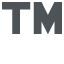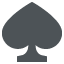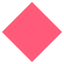108 Coders

# How to do clan tags?##How to do clan tags?

I've been a hacker for about a month and I've got plenty of cool codes for FTB3, but I don't know how to do clan tags.

I found a code called clan tag creator and I assume I need to edit the code based on what tag I want to make, but I don't know how.

If someone could could post a tutorial (or link me to one already on the site, if I didn't see it) that would be great.

zack966

Posts : 5
Join date : 2010-08-30
Age : 23
Location : Greenville, SC

##Re: How to do clan tags?

you dont edt the code ,you edit the HEX to what you want

Triippyshot

Posts : 3
Join date : 2010-08-04

##Re: How to do clan tags?

Yeah, edit the Hex... There are certain hex combos for each letter/ Euro or whatevr you want in it...xXBatmanXx

Posts : 15
Join date : 2010-08-28

##Re: How to do clan tags?

I know what you mean, but what do I edit it to for different things (color, symbols, etc.)

zack966

Posts : 5
Join date : 2010-08-30
Age : 23
Location : Greenville, SC

##Re: How to do clan tags?

A=41 a=61 0=30
B=42 b=62 1=31
C=43 c=63 2=32
D=44 d=64 3=33
E=45 e=65 4=34
F=46 f=66 5=35
G=47 g=67 6=36
H=48 h=68 7=37
I=49 i=69 8=38
J=4A j=6A 9=39
K=4B k=6B
L=4C l=6C
M=4D m=6D
N=4E n=6E
O=4F o=6F
P=50 p=70
Q=51 q=71
R=52 r=72
S=53 s=73
T=54 t=74
U=55 u=75
V=56 v=76
W=57 w=77
X=58 x=78
Y=59 y=79
Z=5A z=7ACode:
Symbols: Cap Euro Letters: Lower Euro Letters:
[SPACE]=20 À=c0c3 à=a0c3
!=21 Á=c1c3 á=a1c3
"=22 Â=c2c3 â=a2c3
#=23 Ã=c3c3 ã=a3c3
\$=24 Ä=c4c3 ä=a4c3
%=25 Å=c5c3 å=a5c3
&=26 Æ=c6c3 æ=a6c3
'=27 Ç=c7c3 ç=a7c3
(=28 È=c8c3 è=a8c3
)=29 É=c9c3 é=a9c3
*=2A Ê=cac3 ê=aac3
+=2B Ë=cbc3 ë=abc3
,=2C Ì=ccc3 ì=acc3
.=2E Î=cec3 î=aec3
/=2F Ï=cfc3 ï=afc3
:=3A Ñ=d1c3 ñ=b1c3
;=3B Ò=d2c3 ò=b2c3
<=3C Ó=d3c3 ó=b3c3
==3D Ô=d4c3 ô=b4c3
>=3E Õ=d5c3 õ=b5c3
?=3F Ö=d6c3 ö=b6c3
@=40 Ø=d8c3 ø=b8c3
[=5B Ù=d9c3 ù=b9c3
\=5C Ú=dac3 ú=bac3
]=5D Û=dbc3 û=bbc3
^=5E Ü=dcc3 ü=bcc3
_=5F Ÿ=b8c5 ÿ=bfc3
`=60 ß=9fc3
{=7B
|=7C
}=7D
~=7E
™=A284E2
¡=A1
®=AE
«=AB
°=B0
»=BB
¿=BF
#N=4E23
{*0}=7D302A7B
{*1}=7D312A7B
{*2}=7D322A7B
{tri}=7D6972747B
{dUP}=7D5055647B
{dDown}=7D6E776F44647B
{dRight}=7D7468676952647B
{dLeft}=7D7466654C647B
{Circ}=7D637269437B
{X}=7D587B
{sq}=7D71737B
{Select}=7D7463656C65537B
{Start}=7D747261747537B
{L1}=7D314C7B
{R1}=7D31527B
{Stick}=7D6B636974537B
Code:
Definitions:
{*0} = white
{*1} = red
{*2} = yellow
#N = next line
{tri} = Triangle
{Circ} = Circle
{X} = Cross
{sq} Square
{Select} = SELECT
{Start} = START
{L1} = Left Trigger
{R1} = Right Trigger
{Stick} = Analogue Stick

_________________
-_-_-_-_-_-_-_-_-_-_-_-_-_-_-|MIPSoder
<(*-*)>mprt17Posts : 73
Join date : 2010-08-04
Age : 29
Location : cyberspace##Re: How to do clan tags?

^ Oh, yeaaaa I remember that... I can't remember all those hex's all at once lol. Thanx.xXBatmanXx

Posts : 15
Join date : 2010-08-28

##Re: How to do clan tags?

np

_________________
-_-_-_-_-_-_-_-_-_-_-_-_-_-_-|MIPSoder
<(*-*)>mprt17Posts : 73
Join date : 2010-08-04
Age : 29
Location : cyberspace##Re: How to do clan tags?

Everybody asks me to do clan tags now, lol..xXBatmanXx

Posts : 15
Join date : 2010-08-28

##Re: How to do clan tags?

sorry i have to ask this, but once i edit the hex, how do i use it, because it just freezes until i turn it off and if i turn it on outside of "edit clan tag" my psp freezes.

zack966

Posts : 5
Join date : 2010-08-30
Age : 23
Location : Greenville, SC

##Re: How to do clan tags?

Nevermind i got it to work.

Thanks for the help!

zack966

Posts : 5
Join date : 2010-08-30
Age : 23
Location : Greenville, SC

##Re: How to do clan tags?